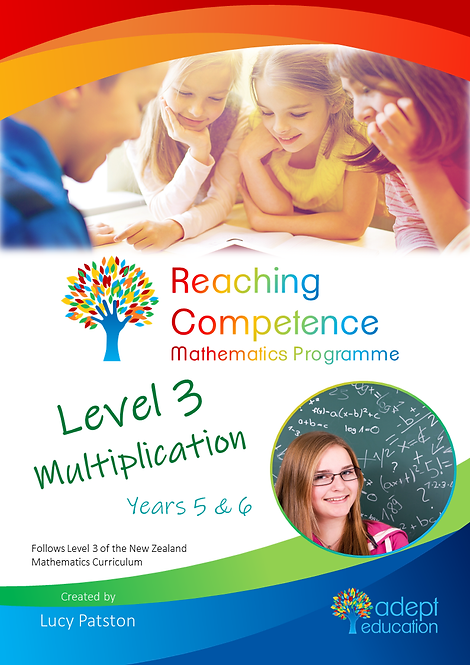`Level 3 Multiplication covers the multiplication only learning intentions from the Linear Series for children aged about 9 to 11 years old (in Years 5 and 6). At this level, students should have committed most of their times tables (to 12 x 12) to memory and understand the fundamental concept of multiplication. They should now be gaining awareness of the relationship between multiplication and division and be starting to develop the ability to extend their core strategies for addition and subtraction to multiplication (and later division). From here, students have a base knowledge to grasp algebra. Get this 92-page activity book in PDF format immediately upon purchase.`

# Level 3 Multiplication e-Copy

\$7.95Price
• Level 3 Multiplication - taken from the Reaching Competence Mathematics Programme.

At this level, students should have committed most of their times tables (to 12 x 12) to memory and understand the fundamental concept of multiplication. They should now be gaining awareness of the relationship between multiplication and division and be starting to develop the ability to extend their core strategies for addition and subtraction to multiplication (and later division). From here, students have a base knowledge to grasp algebra.

You will also get: